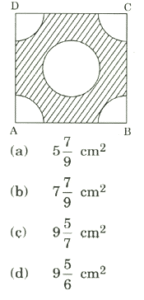# CDS 2018 Maths Question Paper -2

1. The highest four-digit number which is divisible by each of the numbers 16, 36, 45, 48 is

(a) 9180

(b)          9360

(c)           9630

(d)          9840

1. If x = ya, y = zb and = xc, then the value of abc is

(a) 1

(b) 2

(c) -1

(d) 0

1. If x = 2 + 22/3 + 21/3, then the value of the expression x3 – 6×2 + 6x will be

(a) 2

(b) 1

(c) 0

(d) -2

1. How many five-digit numbers of the form XXYXX is/are divisible by 33 ?

(a)          1

(b)          3

(c)           5

(d)          Infinite

1. A five-digit number XY235 is divisible by 3 where x and Y are digits satisfying X + Y ≤ 5. What is the number of possible pairs of values of (X, Y) ?

(a) 5

(b) 6

(c) 7

(d) 9

1. If x2 — 6x – 27 > 0, then which one of the following is correct ?

(a)          – 3 < x < 9

(b)          x < 9 or x > – 3

(c)          x>9orx<-3

(d)          x < – 3 only

1. The number of divisors of the number 38808, exclusive of the divisors 1 and itself, is

(a)          74

(b)          72

(c)           70

(d)          68

1. HCF and LCM of two polynomials are (x + 3) and (x3 – 9×2 — x + 105) respectively. If one of the two polynomials is x2 – 4x – 21, then the other is

(a)          x2 + 2x – 21

(b)          x2 + 2x + 15

(c)           x2 – 2x – 15

(d)          x2 – x – 15

1. If α and β are two real numbers such that α + β = q/p and α β = r/p, where 1 < p < q < r, then which one of the following is the greatest ?

(a) 1/ α + β

(b) 1/ α +1/ β

(c) -1/ α β

(d) α β/ α + β

1. Two workers ‘A’ and ‘B’ working together completed a job in 5 days. Had ‘A’ worked twice as efficiently as he actually did and ‘B’ worked one-third as efficiently as he actually did, the work would have completed in 3 days. In how many days could ‘A’ alone complete the job ?

(a) 3 ½ days

(b) 4 1/6 days

(c) 5 ½ days

(d) 6 ¼ days

1. If x6 _1/x6 = k (x2+1/x2), then k is equal to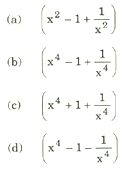1. If the sum of the squares of three consecutive natural numbers is 110, then the sum of their

cubes is

(a)          625

(b)          654

(c)           684

(d)          725

1. The product of two integers p and q, where p > 60 and q > 60, is 7168 and their HCF is 16. The sum of these two integers is

(a)          256

(b)          184

(O           176

(d)          164

1. If log102 = 0.3010 and log103 = 0.4771, then the value of log100 ( 0.72) is equal to

(a)          0.9286

(b)          1.9286

(c)           1.8572

(d)          1.8572

1. If ax = by = cz and abc = 1, then the value of 1/x +1/y+ 1/z will be equal to

(a)          -1

(b)          0

(c)           1

(d)          3

1. If α and β are the roots of the equation ax2 + bx + c = 0, then the value of 1/aα +b +1/a β +b is

(a) a/bc

(b) b/ac

(c) c/ab

(d) 1/abc

1. Consider the following statements in respect of three 3-digit numbers XYZ, YZX and ZXY :
2. The sum of the numbers is not divisible by (X + Y + Z).
3. The sum of the numbers is divisible by 111.

Which of the above statements is/are correct ?

(a)          1 only

(b)          2 only

(c)           Both 1 and 2

(d)          Neither 1 nor 2

1. The number of all pairs (m, n), where m and n are positive integers, such that 1/m +1/n -1/mn = 2/5 is

(a)          6

(b)          5

(c)           4

(d)          2

1. If a = xyP-1, b = yzq-1, c = zxr-1, then aq – r br – p cp-q is equal to

(a)          abc

(b)          xyz

(c)           0

(d)          None of the above

1. The number of sides of two regular polygons are in the ratio 5 : 4. The difference between their interior angles is 9°. Consider the following statements :
2. One of them is a pentagon and the other is a rectangle.
3. One of them is a decagon and the other is an octagon.
4. The sum of their exterior angles is 720°.

Which of the above statements is/are correct ?

(a)          1 only

(b)          2 only

(c)           1 and 3

(d)          2 and 3

1. The minimum value of the expression 2×2 +5x + 5 is

(a)          5

(b)          15/8

(c) – 15/8

(d)          0

1. If H is the harmonic mean of P and Q, then the value of H/P + H/Q is

(a)          1

(b)          2

(c) P+Q/PQ

(d) PQ/P+Q

1. The sum of all possible products taken two at a time out of the numbers ± 1, ± 2, ± 3, + 4 is

(a)          0

(b)          – 30

(c)           30

(d)          55

1. The remainder when 3×3 – 2x2y – 13xy2 + 10y3 is divided by (x – 2y) is equal to

(a)          Zero

(b)          y

(c)           y-5

(d)          y + 3

1. If ab + be + ca = 0, then the value of (b2 – ca) (c2 – ab) + (a2 – bc) (c2 – ab) + (a2 – bc) (b2 – ca)/(a – bc) (b – ca) (c – ab) is

(a) -1

(b) 0

(c) 1

(d) 2

1. What is the principal amount which earns Rs.210 as compound interest for the second year at 5% per annum ?

(a) Rs.2000

(b) Rs.3200

(c) Rs.4000

(d) Rs.4800

1. In an examination, 50% of the candidates failed in English, 40% failed in Hindi and 15% failed in both the subjects. The percentage of candidates who passed in both English and Hindi is

(a)          20%

(b)          25%

(c)           60%

(d)          75%

1. A train 100 m long passes a platform 100 m long in 10 seconds. The speed of the train is

(a)          36 kmph

(b)          45 kmph

(c)           54 kmph

(d)          72 kmph

1. A cyclist covers his first 20 km at an average speed of 40 kmph, another 10 km at an average speed of 10 kmph and the last 30 km at an average speed of 40 kmph. Then the average speed of the entire journey is

(a)          20 kmph

(b)          26.67 kmph

(c)           28-24 kmph

(d)          30 kmph

1. In a race of 1000 m, A beats B by 150 m, while in another race of 3000 m, C beats D by 400 m. Speed of B is equal to that of D. (Assume that A, B, C and D run with uniform speed in all the events). If A and C participate in a race of 6000 m, then which one of the following is correct ?

(a)          A beats C by 250 m

(b)          C beats A by 250 m

(c)           A beats C by 115.38 m

(d)          C beats A by 115-38 m

1. The sum of ages of a father, a mother, a son Sonu and daughters Savita and Sonia is 96 years. Sonu is the youngest member of the family. The year Sonu was bom, the sum of the ages of all the members of the family was 66 years. If the father’s age now is 6 times that of Sonu’s present age, then 12 years hence, the father’s age will be

(a)          44 years

(b)          45 years

(c)           46 years

(d)          48 years

32.‘A’ is thrice as good a workman as ‘B’ and takes 10 days less to do a piece of work than ‘B’ takes. The number of days taken by ‘B’ alone to finish the work is

(a)          12

(b)          15

(c)           20

(d)          30

1. Out. of 85 children playing badminton or table tennis or both, the total number of girls in the group is 70% of the total number of boys in the group. The number of boys playing only badminton is 50% of the number of boys and the total number of boys playing badminton is 60% of the total number of boys. The number of children playing only table tennis is 40% of the total number of children and a total of 12 children play badminton and table tennis both. The number of girls playing only badminton is

(a)          14

(b)          16

(c)           17

(d)          35

1. A person bought two articles X and Y from a departmental store. The sum of prices before sales tax was Rs.130. There was no sales tax on the article X and 9% sales tax on the article Y. The total amount the person paid, including the sales tax was Rs.136.75. What was the price of the article Y before sales tax ?

(a) Rs.75

(b) Rs.85

(c) Rs.122

(d) Rs.125

1. According to Mr. Sharma’s will, half of his property goes to his wife and the rest is equally divided between his two sons, Ravi and Raj. Some years later, Ravi dies and leaves half of his property to his widow and rest to his brother Raj. When Raj dies he leaves half of his property to his widow and remaining to his mother, who is still alive. The mother now owns Rs.88,000 worth of the

property. The total worth of the property of Mr. Sharma was

(a) Rs.1,00,000

(b) Rs.1,24,000

(c) Rs.1,28,000

(d) Rs.1,32,000

1. X bought 4 bottles of lemon juice and Y bought one bottle of orange juice. Orange juice per bottle costs twice the cost of lemon juice per bottle. Z bought nothing but contributed ? 50 for his share of the drink which they mixed together and shared the cost equally. If Z’s Rs.50 is covered from his share, then what is the cost of one bottle of orange juice ?

(a) Rs.75

(b) Rs.50

(c) Rs.46

(d) Rs.30

1. Ten (10) years before, the ages of a mother and her daughter were in the ratio 3 : 1. In another 10 years from now, the ratio of their ages will be 13 : 7. What are their present ages?

(a)          39 years, 21 years

(b)          55 years, 25 years

(c)           75 years, 25 years

(d)          49 years, 31 years

1. In a class of 60 boys, there are 45 boys who play chess and 30 boys who play carrom. If every boy of the class plays at least one of the two games, then how many boys play carom only?

(a)          30

(b)          20

(c)           15

(d)          10

1. Two equal amounts were borrowed at 5% and 4% simple interest. The total interest after

4 years amounted to Rs.405. What was the total amount borrowed ?

(a) Rs.1075

(b) Rs.1100

(c) Rs.1125

(d) Rs.1150

1. Twelve (12) men work 8 hours per day and require 10 days to build a wall. If 8 men are available, how many hours per day must they work to finish the work in 8 days ?

(a)          10 hours

(b)          12 hours

(c)           15 hours

(d)          18 hours

1. A milk vendor bought 28 litres of milk at the rate of W 8.50 per litre. After adding some water he sold the mixture at the same price. If his gain is 12.5%, how much water did he add?

(a)          4.5 litres

(b)          4 litres

(c)           3.5 litres

(d)          3 litres

1. The minute hand of a clock overtakes the hour hand after every 72 minutes of correct time. How much time does the clock lose or gain in a day of normal time ?

(a)          Lose 121 9/11 minutes

(b) Lose 1571/11 minutes

(c)           Gain 121 9/11 minutes

(d)          Gain 157 1/11 minutes

1. A thief steals a car parked in a house and goes away with a speed of 40 kmph. The theft was discovered after half an hour and immediately the owner sets off in another car with a speed of 60 kmph. When will the owner meet the thief ?

(a)          55 km from the owner’s house and one hour after the theft

(b)          60 km from the owner’s house and 15 hours after the theft

(c)           60 km from the owner’s house and 1-5 hours after the discovery of the theft

(d)          55 km from the owner’s house and 1-5 hours after the theft

1. X and Y together can finish a job in 6 days. X can alone do the same job in 12 days. How long will Y alone take to do the same job ?

(a)          16 days

(b)          12 days

(c)           10 days

(d)          8 days

1. Twelve (12) persons can paint 10 identical rooms in 16 days. In how many days can 8 persons paint 20 such rooms ?

(a)          12

(b)          24

(c)           36

(d)          48

1. There are n zeros appearing immediately after the decimal point in the value of (0.2)25. It is given that the value of log10 2 = 0.30103. The value of n is

(a)          25

(b)          19

(c)           18

(d)          17

1. The ratio of the sum and difference of the ages of the father and the son is 11 : 3. Consider the following statements :
2. The ratio of their ages is 8 : 5.
3. The ratio of their ages after the son attains twice the present age will be 11 : 8.

Which of the statements given above is/are correct ?

(a)          1 only

(b)          2 only

(c)           Both 1 and 2

(d)          Neither 1 nor 2

1. The solution of linear inequalities x + y ≥ 5 and x – y ≤ 3 lies

(a)          Only in the first quadrant

(b)          In the first and second quadrants

(c)           In the second and third quadrants

(d)          In the third and fourth quadrants

1. It is given that the equations x2 – y2 = 0 and (x – a)2 + y2 = 1 have single positive solution. For this, the value of ‘a’ is

(a) √2

(b) 2

(c)  -√2

(d) 1

1. If α, β and γ are the zeros of the polynomial f(x) = ax3 + bx2 + cx + d, then α 2 + β 2 + γ 2 is equal to

(a) b2 – ac/a2

(b) b2 – 2ac/a

(c) b2 + 2ac/b2

(d) b2 – 2ac/a2

Consider the following for the next 04 (four) items that follow:

In an examination of Class XII, 55% students passed in Biology, 62% passed in Physics, 60% passed in Chemistry, 25% passed in Physics and Biology, 30% passed in Physics and Chemistry, 28% passed in Biology and Chemistry. Only 2% failed in all the subjects.

1. What percentage of students passed in all the three subjects ?

(a)          6

(b)          5

(c)           4

(d)          3

1. What percentage of students passed in exactly one subject ?

(a)          21

(b)          23

(c)           25

(d)          27

1. If the number of students is 360, then how many passed in at least two subjects ?

(a)          270

(b)          263

(c)           265

(d)          260

1. What is the ratio of number of students who passed in both Physics and Chemistry to number of students who passed in both Biology and Physics but not Chemistry ?

(a)          7 : 10

(b)          10 : 7

(c)           9 : 7

(d)          7 : 9

1. Data on ratings of hotels in a city is measured on

(a)          Nominal scale

(b)          Ordinal scale

(c)           Interval scale

(d)          Ratio scale

1. The average marks of section A are 65 and that of section B are 70. If the average marks of both the sections combined are 67, then the ratio of number of students of section A to that of section B is

(a)          3 : 2

(b)          1 : 3

(c)           3 : 1

(d)          2 : 3

57.The median of 19 observations is 30. Two more observations are made and the values of these are 8 and 32. What is the median of the 21 observations ?

(a)          32

(b)          30

(c)           20

(d)          Cannot be insufficient data determined due to

1. As the number of observations and classes increases, the shape of a frequency polygon

(a)          Tends to become jagged

(b)          Tends to become increasingly smooth

(c)           Stays the same

(d)          Varies only if data become more reliable

1. Let X1 and x2 (where x2 > x1) be the means of two sets comprising n1 and n2 (where n2 < n1) observations respectively. If x is the mean when they are pooled, then which one of the following is correct ?

(a)          X1 < x < x2

(b)          x > x2

(c)           x < x1

(d)          (x1- x) + (x2 – x) = 0

1. Consider the following statements :

Statement I: Median can be computed even when the end intervals of a frequency distribution are open.

Statement II: Median is a positional average.

Which one of the following is correct in respect of the above statements ?

(a)          Both Statement I and Statement II are true and Statement II is the correct explanation of Statement I

(b)          Both Statement I and Statement II are true and Statement II is not the correct explanation of Statement I

(c)           Statement I is true but Statement II is false

(d)          Statement I is false but Statement II is true

1. If cos Ө =1/√5 , where 0 < Ө < π/2, then 2tan Ө /1-tan2 Ө is equal to

(a)          4/3

(b)          – 4/3

(c)           1/3

(d)          – 2/3

1. If 0 < Ө < 90°, 0 < Ø < 90° and cos Ө < cos Ø, then which one of the following is correct ?

(a)          Ө < Ø

(b)          Ө > Ø

(c)           Ө + Ø = 90°

(d)          No conclusion can be drawn

63.On the top of a hemispherical dome of radius r, there stands a flag of height h. From a point on the ground, the elevation of the top of the flag is 30°. After moving a distance d towards the dome, when the flag is just visible, the elevation is 45°. The ratio of h to r is equal to

(a) √2 -1

(b) √3 +1/2√2

(c) √3+1/2√2 d

(d) (√3 + 1) (√2 -1)/2√2 d

1. Let sin (A + B) = √3/2 and cos B = √3/2, where A, B are acute angles. What is tan (2A – B) equal to?

(a) 1/2

(b) √3

(c) 1/√3

(d) 1

1. Consider the following statements :
2. If cos Ө /1-sin Ө + cos Ө /1+sin Ө = 4, where 0 < Ө < 90°, then Ө = 60°.
3. If 3 tan Ө + cot Ө = 5 cosec Ө, where 0 < Ө < 90°, then Ө = 60°.

Which of the statements given above is/are correct ?

(a)          1 only

(b)          2 only

(c)           Both 1 and 2

(d)          Neither 1 nor 2

1. Consider the following statements :
2. cos2 Ө = 1- p2+q2/2pq, where p, q are non-zero real numbers, is possible only when p = q.
3. tan2 Ө = 4pq/(p+q)2-1, where p, q are non-zero real numbers, is possible only when p = q.

Which of the statements given above is/are correct ?

(a)          1 only

(b)          2 only

(c)           Both 1 and 2

(d)          Neither 1 nor 2

1. Consider the following statements :
2. cos Ө + sec Ө can never be equal to 1.5.
3. sec2 Ө + cosec2 Ө can never be less than 4.

Which of the statements given above is/are correct ?

(a)          1 only

(b)          2 only

(c)           Both 1 and 2

(d)          Neither 1 nor 2

1. sin2 x + sin x = 1, then what is the value of cos12 x + 3 cos10 x + 3 cos8 x + cos6 x?

(a)          -1

(b)          0

(c)           1

(d)          8

1. 3 sin Ө + 5 cos Ө = 4, then what is the value of (3 cos Ө -5 sin Ө)2?

(a)          9

(b)          12

(c)           16

(d)          18

1. If cot Ө (1 + sin Ө) = 4m and cot Ө (1 – sin Ө) = 4n, then which one of the following is correct?

(a)          (m2 + n2)2 = mn

(b)          (m2 – n2)2 = mn

(c)           (m2 – n2)2 = m2n2

(d)          (m2 + n2)2 = m2n2

1. If base and hypotenuse of a right triangle are (u2 – v2) and (u2 + v2) respectively and the area of the triangle is 2016 square units, then the perimeter of the triangle may be

(a)          224 units

(b)          288 units

(c)           448 units

(d)          576 units

1. A circle is inscribed in an equilateral triangle of side of length I. The area of any square inscribed in the circle is

(a) l2/2

(b) √3l2/4

(c) l2/4

(d) l2/6

1. Walls (excluding roofs and floors) of 5 identical rooms having length, breadth and height 6 m,

4 m and 2.5 m respectively are to be painted. Out of five rooms, two rooms have one square window each having a side of 2.5 m. Paints are available only in cans of 1 litre; and 1 litre of paint can be used for painting 20 square metres. The number of cans required for painting is

(a) 10

(b) 12

(c) 13

(d) 14

1. Let S be the parallelogram obtained by joining the mid-points of the parallelogram T. Consider the following statements :
2. The ratio of area of T to that of S is 2 : 1.

2              The perimeter of S is half of the sum of diagonals of T.

Which of the above statements is/are correct ?

(a)          1 only

(b)          2 only

(c)           Both 1 and 2

(d)          Neither 1 nor 2

1. The sides of a triangle are 5 cm, 6 cm and 7 cm. The area of the triangle is approximately

(a)          14.9 cm2

(b)          14.7 cm2

(c)           14.5 cm2

(d)          14.3 cm2

1. There is a path of width 5 m around a circular plot of land whose area is 144π m2. The total area of the circular plot including the path surrounding it is

(a)          349 π m2

(b)          289 π m2

(c)           209 π m2

(d) 149 π m2

1. The lateral surface area of a cone is 462 cm2. Its slant height is 35. The radius of the base of the cone is

(a)          8.4 cm

(b)          6.5 cm

(c)           4.2 cm

(d)          3.2 cm

1. A semi-circular plate is rolled up to form a conical surface. The angle between the generator and the axis of the cone is

(a) 60°

(b) 45°

(c) 30°

(d) 15°

79.A solid right cylinder is of height π cm. If its lateral surface area is half its total surface area, then the radius of its base is

(a)          π /2 cm

(b)          π cm

(c)           1/ π cm

(d)          2/ π cm

1. A rectangular block of length 20 cm, breadth 15 cm and height 10 cm is cut up into exact number of equal cubes. The least possible number of cubes will be

(a)          12

(b)          16

(c)           20

(d)          24

1. If the diagonal of a cube is of length I, then the total surface area of the cube is

(a)          3l2

(b)          √3l2

(c)           √2l2

(d)          2I2

1. An equilateral triangle, a square and a circle have equal perimeter. If T, S and C denote the area of the triangle, area of the square and area of the circle respectively, then which one of the following is correct ?

(a)          T < S < C

(b)          S < T < C

(c)           C < S < T

(d)          T < C < S

1. The areas of two similar triangles are (7-4 √3 ) cm2 and (7 + 4√3) cm2 respectively. The ratio of their corresponding sides is

(a)          7-4√3

(b)          7-3√3

(c)           5 – √3

(d)          5+√3

1. The chord of a circle is √3 times its radius. The angle subtended by this chord at the minor arc is k times the angle subtended at the major arc. What is the value of k ?

(a)          5

(b)          2

(c)           1/2

(d)          1/5

1. In a triangle ABC, the sides AB, AC are produced and the bisectors of exterior angles of ∠ABC and ∠ACB intersect at D. If ∠BAC = 50°, then ∠BDC is equal to

(a)          115°

(b)          65°

(c)           55°

(d)          40°

1. Two cones have their heights in the ratio 1:3. If the radii of their bases are in the ratio 3 : 1, then the ratio of their volumes will be

(a)          1: 1

(b)          2 : 1

(c)           3 : 1

(d)          9 : 1

1. If two lines AB and CD intersect at O such that ∠AOC = 5 ∠AOD, then the four angles

at O are

(a)          40°, 40°, 140°, 140°

(b)          30°, 30°, 150°, 150°

(c)           30°, 45°, 75°, 210°

(d)          60°, 60°, 120°, 120°

1. If a point P moves such that the sum of the squares of its distances from two fixed points A and B is a constant, then the locus of the point P is

(a)          A straight line

(b)          A circle

(c)           Perpendicular bisector of AB

(d)          An arbitrary curve

1. If ABC is a right-angled triangle with AC as its hypotenuse, then which one of the following is correct ?

(a)          AC3 < AB3 + BC3

Ob)        AC3 > AB3 + BC3

(c)           AC3 ≤ AB3 + BC3

(d)          AC3 ≥ AB3 + BC3

1. The area of the region bounded externally by a square of side 2 a cm and internally by the

Circle touching the four sides of the square is

(a)          (4 – π)a2

(b)          (π — 2)a2

(c)           (8 – π)a2/2

(d)          (π – 2)a2/2

1. In the figure given below, ABC is a right-angled triangle where ∠A = 90°, AB =s p cm and AC = q cm. On the three sides as diameters semicircles are drawn as shown in the figure. The area of the shaded portion, in square cm, is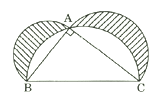(a)          pq

(b)          π (p2 + q2)/2

(c)           π (p2 + q2)

(d)          pq/2

1. In the figure given below, the radius of the circle is 6 cm and AT = 4 cm. The length of tangent PT is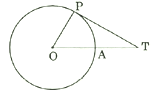(a)          6 cm

(b)          8 cm

(c)           9 cm

(d)          10 cm

1. In the figure given below, ABCD is the diameter of a circle of radius 9 cm. The lengths AB, BC and CD are equal. Semicircles are drawn on AB and BD as diameters as shown in the figure. What is the area of the shaded region ?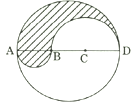(a)          9 π

(b)          27 π

(c)           36 π

(d)          81 π

1. In the figure given below, what is ∠BCD equal to ?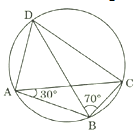(a)          70°

(b)          75°

(c)           80°

(d)          90°

1. In the figure given below, AB is the diameter of the circle whose centre is at O. Given that∠ ECD = ∠EDC = 32°, then ∠CEF and ∠COF respectively are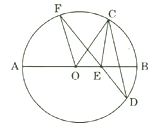(a) 32°, 64°

(b)          64°, 64°

(c)           32°, 32°

(d)          64°, 32°

1. In the figure given below, ΔABR ~ ΔPQR. If PQ = 3 cm, AB = 6 cm, BR = 8.2 cm and PR = 5.2 cm, then QR and AR are respectively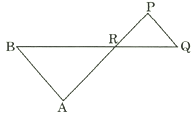(a)          8.2 cm, 10.4 cm

(b)          4.1 cm, 6 cm

(c)           2.6 cm, 5.2 cm

(d)          4.1 cm, 10.4 cm

1. In the figure given below, ABC is a triangle with AB perpendicular to BC. Further BD is perpendicular to AC. If AD = 9 cm and DC = 4 cm, then what is the length of BD ?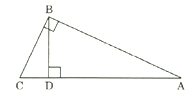(a)          13/36 cm

(b) 36/13 cm

(c)           13/2 cm

(d)          6 cm

1. In the figure given below, the diameter of bigger semicircle is 108 cm. What is the area of the shaded region ?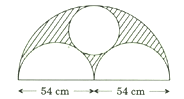(a) 201 π cm2

(b) 186.3 π cm2

(c) 405 π cm2

(d) 769.5 π cm2

1. In the figure given below, ABC is an equilateral triangle with each side of length 30 cm. XY is parallel to BC, XP is parallel to AC and YQ is parallel to AB. If XY + XP + YQ is 40 cm, then the value of PQ is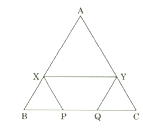(a) 5 cm

(b) 12 cm

(c) 15 cm

(d) 10 cm

1. In the figure given below, ABCD is a square of side 4 cm. Quadrants of a circle of diameter 2 cm are removed from the four corners and a circle of diameter 2 cm is also removed. What is the area of the shaded region ?